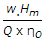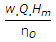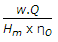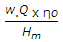# Mechanical Engineering - Hydraulic Machines

### Exercise :: Hydraulic Machines - Section 2

1.

The impeller of a centrifugal pump may have

 A. volute casing B. volute casing with guide blades C. vortex casing D. any one of these

Explanation:

No answer description available for this question. Let us discuss.

2.

Kinematic similarity is said to exist between the model and the prototype, if both of them

 A. have identical velocities B. are equal in size and shape C. are identical in shape, but differ only in size D. have identical forces

Explanation:

No answer description available for this question. Let us discuss.

3.

Power required (in watts) to drive a centrifugal pump is (where Hm = Manometric head in metres, w = Specific weight in N/m3, Q = Discharge of the pump in m3/s, and ηo = Overall efficiency of the pump)

 A.B.C.D.Explanation:

No answer description available for this question. Let us discuss.

4.

A reciprocating pump is suitable for less discharge and higher heads.

 A. True B. False

Explanation:

No answer description available for this question. Let us discuss.

5.

The flow ratio of Francis turbine is defined as the ratio of the

 A. velocity of flow at inlet to the theoretical jet velocity B. theoretical velocity of jet to the velocity of flow at inlet C. velocity of runner at inlet to the velocity of flow at inlet D. none of the above